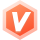sasigeinaruto 2022-05-13 10:05 采纳率: 75%浏览 88

# c++类编程学习问题

（1）能通过对“>>”、“<<”的重载实现数组元素的直接输入及其输出。输入时，第一次输入的值为数组长度，后面连续输入若干个数作为数组内容。输出时，首先提示该数组元素个数，然后依次输出该数组各元素；

（2）重载“=”、“+”、“-” 运算符使之能对两个数组类对象进行直接赋值、加减运算。

（3）写出主函数对该类进行测试。要求：

1）先构造包含10个数组元素的数组对象a，再调用流提取运算符重载函数实现a的元素初始化（元素值分别为1—10），输出a数组；

2）并利用a来构造数组b，输出数组b，并调用get函数获取b中下标为奇数的各元素值并输出，然后调用set函数将上述元素值乘以2，输出b数组；

3）构造一个包含5个元素的数组c，其值全为1，输出该数组，再调用ReSize函数将其大小重置为10，调用“=”重载函数将a的值复制给c，输出数组c；

4）分别将b+c，b-c的值送给数组d和数组e，并输出结果。

class intArray

{public:

intArray(int size);//构造函数

intArray(const intArray &x);//复制构造函数

~intArray();//析构函数

bool Set(int i, int elem);//设置第i个数组元素的值，设置成功返回true，失败返回false

bool Get(int i, int &elem); //获取第i个数组元素的值，获取成功返回true，失败返回false

int Length( ) const;//获取数组的长度

void ReSize ( int size ); //重置数组

intArray &operator=(const intArray &other); //赋值运算符“=”重载函数

intArray &operator+(const intArray &other); //加运算符“=”重载函数

intArray &operator-(const intArray &other) ; //减运算符“=”重载函数

friend ostream & operator>>(ostream &, intArray &); //数组的整体输入

friend ostream & operator<<(ostream &, intArray &); //数组的整体输出

private:

int *element; //指向动态数组的指针

int arraysize; //数组的当前长度

};

• 写回答

#### 1条回答默认 最新

•吕布辕门 后端领域新星创作者 2022-05-13 10:34
关注

可以参考一下！亲

``````
#include <iostream>
#include<string>
using namespace std;
class intArray
{
friend istream& operator>>(istream& cin, intArray&);//数组的整体输入
friend ostream& operator<<(ostream& cout, intArray&);//数组的整体输出
public:
intArray(){}
intArray(int size);//构造函数
intArray(const intArray& x);//复制构造函数
~intArray();//析构函数
bool Set(int i, int elem)//设置第i个数组元素的值，设置成功返回true，失败返回false
{
if (i>=0&&i<this->arraysize)
{
this->element[i] = elem;
return true;
}
else
{
return false;
}
}
int Get(int i)//获取第i个数组元素的值
{
if (i > 0 && i < this->arraysize)
{
return this->element[i];
}
}
int Length() {//获取数组长度
return arraysize;
}

void ReSize(int size);//重置数组的长度
intArray& operator=(const intArray& other);//赋值运算符“=”重载函数
intArray operator+(const intArray& other);//加运算符“+”重载函数
intArray operator-(const intArray& other);//减运算符“-”重载函数

private:
int* element;//指向动态数组的指针
int arraysize;//数组的当前长度
};

intArray::~intArray() {
delete element;
element = NULL;
}
intArray::intArray(const intArray& x) {
this->arraysize = x.arraysize;
this->element = new int[arraysize];
for (int i = 0; i < arraysize; i++)
{
this->element[i] = x.element[i];
}
}
intArray::intArray(int size) {
this->arraysize = size;
this->element = new int[size];
}

/*
对数组对象进行输入
*/
istream& operator>>(istream& cin, intArray& arr) {
int temp = 0;
for (int i = 0; i <arr.arraysize; i++)
{
cin >> temp;
arr.element[i] = temp;
}
return cin;
}
/*
对数组对象进行输出
*/
ostream& operator<<(ostream& cout, intArray& arr) {
for (int i = 0; i < arr.arraysize; i++)
{
cout << arr.element[i] << endl;
}
return cout;
}
intArray& intArray::operator=(const intArray& other)//赋值运算符“=”重载函数
{
if (this->element != NULL)
{
delete this->element;
this->element = NULL;
}
this->arraysize = other.arraysize;
this->element = new int[arraysize];
for (int i = 0; i < arraysize; i++)
{
this->element[i] = other.element[i];
}
return *this;
}
intArray intArray:: operator+(const intArray& other)//加运算符“+”重载函数
{
intArray temp(this->arraysize);
for (int i = 0; i < this->arraysize; i++)
{
temp.element[i] = this->element[i] + other.element[i];
}
return temp;
}
intArray intArray:: operator-(const intArray& other)//减运算符“-”重载函数
{
intArray temp(this->arraysize);
for (int i = 0; i < this->arraysize; i++)
{
temp.element[i] = this->element[i] - other.element[i];
}
return temp;
}
void intArray::ReSize(int size) {
this->arraysize = size;
if (this->element!=NULL)
{
delete this->element;
}
this->element = new int[size];
}
intArray& testA() {
int size = 0;
cout << "请输入要构造的数组长度：" << endl;
cin >> size;
intArray* a=new intArray(size);
cout << "请输入" << size << "个数字来为数组对象a初始化：" << endl;
cin >> *a;
cout << "=================打印数组对象a===============" << endl;
cout << *a;
return *a;
}
intArray& testB(intArray& a) {
//拷贝构造数组b
intArray* b = new intArray(a);
int size = a.Length();//获取数组长度
cout << "===============打印数组对象b=================" << endl;
cout << *b << endl;
cout << "==========打印数组对象b下标为奇数的值==========" << endl;
for (int i = 1; i < size; i += 2)
{
cout << (*b).Get(i) << endl;
}
cout << "=======将奇数下标的值翻倍，再打印数组对象b======" << endl;
for (int i = 1; i < size; i += 2)
{
(*b).Set(i, (*b).Get(i) * 2);
}
cout << *b << endl;
return *b;
}
intArray& testC(intArray& a) {
//构造数组对象c
intArray* c=new intArray(5);
for (int i = 0; i < 5; i++)
{
(*c).Set(i, 1);
}
cout << "===============打印数组对象c=================" << endl;
cout << *c << endl;
(*c).ReSize(10);
(*c) = a;
cout << "===========打印赋值操作后的数组对象c===========" << endl;
cout << *c << endl;
return *c;
}
void testD(intArray& b, intArray& c) {
intArray d = b + c;
intArray e = b - c;
cout << "================打印数组对象d=================" << endl;
cout << d << endl;
cout << "================打印数组对象e================" << endl;
cout << e << endl;
}
void test() {
cout << "*************问题1解答*************" << endl << endl;
intArray a=testA();
cout << "*************问题2解答*************" << endl << endl;
intArray b = testB(a);
cout << "*************问题3解答*************" << endl << endl;
intArray c= testC(a);
cout << "*************问题4解答*************" << endl << endl;
testD(b, c);
}
int main()
{
test();
system("pause");
return 0;
}
``````
本回答被题主选为最佳回答 , 对您是否有帮助呢?
评论 编辑记录

#### 悬赏问题

• ¥15 matlab+波形匹配算法
• ¥15 转录组分析做聚类树图时癌旁组被分到了癌组
• ¥15 大一Python字典
• ¥15 multisim电路设计（相关搜索：设计报告）
• ¥15 PC-lint Plus
• ¥15 gpl24676注释
• ¥15 php5.3内存泄露
• ¥15 DigSilent如何复制复合模型到自己案例？
• ¥15 求日版华为b610s-77a 官方公版固件，有偿
• ¥15 关于#java#的问题，请各位专家解答！（相关搜索：java程序）